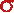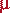Math & Science Home | Proficiency Tests | Mathematical Thinking in Physics | Aeronauts 2000

 CONTENTS Introduction Fermi's Piano Tuner Problem How Old is Old? If the Terrestrial Poles were to Melt... Sunlight Exerts Pressure Falling Eastward What if an Asteroid Hit the Earth Using a Jeep to Estimate the Energy in Gasoline How do Police Radars really work? How "Fast" is the Speed of Light? How Long is a Light Year? How Big is a Trillion? "Seeing" the Earth, Moon, and Sun to Scale Of Stars and Drops of Water If I Were to Build a Model of the Cosmos... A Number Trick Designing a High Altitude Balloon Pressure in the Vicinity of a Lunar Astronaut Space Suit due to Outgassing of Coolant Water Calendar Calculations Telling Time by the Stars - Sidereal Time Fields, an Heuristic Approach The Irrationality ofThe Irrationality ofThe Number (i)i Estimating the Temperature of a Flat Plate in Low Earth Orbit Proving that (p)1/n is Irrational when p is a Prime and n>1 The Transcendentality ofIdeal Gases under Constant Volume, Constant Pressure, Constant Temperature and Adiabatic Conditions Maxwell's Equations: The Vector and Scalar Potentials A Possible Scalar Term Describing Energy Density in the Gravitational Field A Proposed Relativistic, Thermodynamic Four-Vector Motivational Argument for the Expression-eix=cosx+isinx Another Motivational Argument for the Expression-eix=cosx+isinx Calculating the Energy from Sunlight over a 12 hour period Calculating the Energy from Sunlight over actual full day Perfect Numbers-A Case Study Gravitation Inside a Uniform Hollow Sphere Further note on Gravitation Inside a Uniform Hollow Sphere Pythagorean Triples Black Holes and Point Set Topology Additional Notes on Black Holes and Point Set Topology Field Equations and Equations of Motion (General Relativity) The observer in modern physics A Note on the Centrifugal and Coriolis Accelerations as Pseudo Accelerations - PDF File On Expansion of the Universe - PDF File

## Sunlight Exerts Pressure

Problem: Estimate the pressure exerted by sunlight on objects at 1 AU from the sun. Discuss the result.

Solution: A dimensional analysis of the solar constant (in units of W/m2) leads rather directly to an intuitive expression for pressure exerted on objects by sunlight.

W/m2 = j/(m2 sec) = (nt m)/(m2 sec) = (nt/m2)(m/sec)

The last term on the right appears to be made up of a pressure and a velocity which, in this case, must be taken to be the velocity of light. Thus, if= solar constant, then/c must be the corresponding pressure3. At 1 AU,= 1.36 x 103 W/m2. The corresponding pressure must be

p = 4.53 x 10-6 nt/m2

(about 6.56 x 10-10 psi).

Now, with this value in hand, let us consider the forces on a 1diameter particle with a density of 1 gm/cm3, such as might be found in the tail of a comet. The projected area, A, of the 1particle is 7.85 x 10-13 m2, and its mass, m, is 5.24 x 10-13 gm = 5.24 x 10-16 kg. The force exerted by sunlight on this particle is

f = pA = 3.56 x 10-18 nt

and its acceleration away from the sun, due to the pressure of sunlight, is

a = 6.79 x 10-3 m/sec2.

The acceleration due to the sun's gravity at 1 AU is

gsun = 5.92 x 10-3 m/sec2

so that the pressure of sunlight overwhelms the solar gravity by a factor4 of

6.79/5.92 = 1.15.

We see here why a comet's tail points away from the sun!

In fact, the ratio of the two accelerations is independent of the distance from the sun for any given particle. The solar constant at a distance l from the sun isSBTs4(Rs/l)2

whereSB is the Stefan-Boltzmann constant (= 5.67 x 10-8 W/(m2K)4), Ts is the sun's surface temperature, and Rs is the solar radius. The corresponding pressure of sunlight at the distance l is

(SBTs4/c)(Rs/l)2.

Now, let a be the acceleration due to light pressure at distance l, and gs be the solar gravitation at the same distance. For a particle of cross sectional area A and mass m, the acceleration, a, is

a = (ASBTs4/mc)(Rs/l)2.

If gso is the solar surface gravity, then

gs = gso(Rs/l)2,

and a/gs = ASBTs4/mcgso.

This last expression is the ratio of the accelerations sought. N.B., The ratio is independent of distance.

As a check on our previous calculation, we use the values:

A = 7.85 x 10-13 m2SB = 5.67 x 10-8 W/(m2K4)
Ts = 5800 °K
m = 5.24 x 10-16 kg
c = 3 x 108 m/sec
gso = 2.74 x 102 m/sec2

With these values, we find a/gs = 1.17, in agreement with our previous result.

Having done the calculation for a 1diameter particle, let us now repeat it for the Earth. For Earth, r = 6.37 x 103 km and m = 5.98 x 1024 kg, and the ratio5 a/gs comes out to

1.63 x 10-14

The perturbation of the Earth due to the pressure of sunlight is too small to detect by any ordinary means.

4 This value is correct for photons that are absorbed. For photons that are reflected, this value must be multiplied by 2.

5 See footnote 4.

Please send suggestions/corrections to:
Web Related: David.Mazza@grc.nasa.gov
Technology Related: Joseph.C.Kolecki@grc.nasa.gov
Responsible NASA Official: Theresa.M.Scott (Acting)